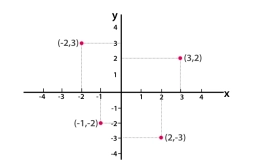# Properties of eq2 roots

If p and q are the zeroes of the quadratic polynomial f(x) = 2x2 - 7x + 3, find the value of p + q - pq.

v =  2

### Step-by-step explanation:Did you find an error or inaccuracy? Feel free to write us. Thank you!

Tips for related online calculators
Are you looking for help with calculating roots of a quadratic equation?
Do you have a linear equation or system of equations and looking for its solution? Or do you have a quadratic equation?